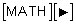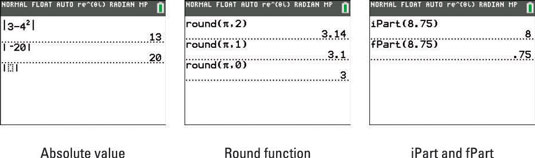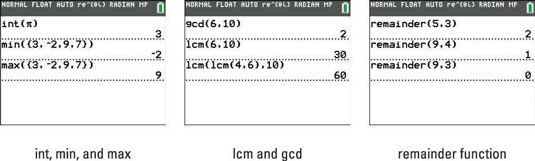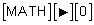##### TI-84 Plus CE Graphing Calculator For Dummies, 3rd EditionOn the TI-84 Plus calculator, several important items are housed in the Math NUM submenu. To access the Math NUM submenu, you press the following keys:## Finding the absolute value with the TI-84 Plus

The abs template evaluates the absolute value of the number or arithmetic expression. Insert the abs template, type an expression, and press [ENTER]. An example of using the abs function is illustrated in the first screen.

The abs template can also be found in the shortcut menu by pressing [ALPHA][WINDOW].## Rounding numbers with the TI-84 Plus calculator

The round function rounds a number or arithmetic expression to a specified number of decimal places. The number or expression to be rounded and the specified number of decimal places are placed after the function separated by a comma and surrounded by parentheses. The calculator supplies the opening parenthesis; you must supply the closing parenthesis. An example of using the round function is the second screen.

## Finding the integer and fractional parts of a value on the TI-84 Plus

Although iPart may sound like the newest Apple product, it’s actually a math function! The iPart and fPart functions (respectively) find the integer and fractional parts of the number, or the arithmetic expression that follows the function. This number or expression must be enclosed in parentheses. The calculator supplies the opening parenthesis; you must supply the closing parenthesis. An example of using the iPart function is the third screen.

## Using the TI-84 Plus calculator's greatest-integer function

The int function finds the largest integer that is less than or equal to the number or arithmetic expression that follows the function. This number or expression must be enclosed in parentheses. The calculator supplies the opening parenthesis; you must supply the closing parenthesis. See the first line in the first screen.## Finding minimum and maximum values in a list of numbers on the TI-84 Plus

The min and max functions find (respectively) the minimum and maximum values in the list of numbers that follows the function. Braces must surround the list, and commas must separate the elements in the list. You can access the braces on the calculator by pressing [2nd][(] and [2nd][)].

The list must be enclosed in parentheses. The calculator supplies the opening parenthesis; you must supply the closing parenthesis. See the last two lines in the first screen.

When using the min or max function to find the minimum or maximum of a two-element list, you can omit the braces that surround the list. For example, min(2, 4) returns the value 2.

## Finding the least common multiple and greatest common divisor

The lcm and gcd functions find (respectively) the least common multiple and the greatest common divisor of the two numbers that follow the function. These two numbers must be separated by a comma and surrounded by parentheses. The calculator supplies the opening parenthesis; you must supply the closing parenthesis. Notice, the second screen also demonstrates how to find the lcm of three numbers.

## Finding the remainder on the TI-84 Plus calculator

The remainder function finds the remainder resulting from dividing two positive whole numbers. The divisor cannot be zero. Pressto insert the remainder function. Enter the dividend and press [,]. Finally, enter the divisor and press [ENTER]. See the third screen.